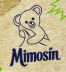## Perl weekly challenge 093

This is a Raku answer for the 093 Perl Weekly Challenge . This is also my first post.

Exercise 1

Using a similar approach than James Curtis-Smith , the solution looks at points with equal slope to see if they are in a straight line. Being less literate in Raku, using classes help me to organize coding ideas.

This exercise gives me the opportunity to work with the type BagHash. The highest value of the slopes stored in a BagHash gives the number of points in a straight line. Happily, the first example in Raku documentation is for a Class Point, an example reused in this solution.

Exercise 2

Contrary to James Curtis-Smith, I really struggled with this exercise. This is probably because of the approach taken.

The binary tree has been defined as 'lines of nodes', with 0 if the node is empty. Example:

While this is an exhaustive definition of the tree, it might not be so convenient for a simple iterable solution. The solution presented involves:

• The definition of the class Tree that defines the levels and the array of lines.
• The definition of two methods that gives the tree line and the tree node.
• A subroutine that iterates through the nodes in the last line to build the tree paths.
• Using the properties of binary trees:
• The number of elements in a level = level.exp(2).
• The position of the previous node of a node = log2( previous node ).round(1)I teach on Social Psychology and have fun in my spare time coding in Raku. "The past is written, the future is left for us to code"